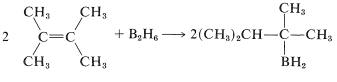# 18.4: Reactions at the Carbonyl Carbon of Carboxylic Acids

$$\newcommand{\vecs}{\overset { \rightharpoonup} {\mathbf{#1}} }$$ $$\newcommand{\vecd}{\overset{-\!-\!\rightharpoonup}{\vphantom{a}\smash {#1}}}$$$$\newcommand{\id}{\mathrm{id}}$$ $$\newcommand{\Span}{\mathrm{span}}$$ $$\newcommand{\kernel}{\mathrm{null}\,}$$ $$\newcommand{\range}{\mathrm{range}\,}$$ $$\newcommand{\RealPart}{\mathrm{Re}}$$ $$\newcommand{\ImaginaryPart}{\mathrm{Im}}$$ $$\newcommand{\Argument}{\mathrm{Arg}}$$ $$\newcommand{\norm}{\| #1 \|}$$ $$\newcommand{\inner}{\langle #1, #2 \rangle}$$ $$\newcommand{\Span}{\mathrm{span}}$$ $$\newcommand{\id}{\mathrm{id}}$$ $$\newcommand{\Span}{\mathrm{span}}$$ $$\newcommand{\kernel}{\mathrm{null}\,}$$ $$\newcommand{\range}{\mathrm{range}\,}$$ $$\newcommand{\RealPart}{\mathrm{Re}}$$ $$\newcommand{\ImaginaryPart}{\mathrm{Im}}$$ $$\newcommand{\Argument}{\mathrm{Arg}}$$ $$\newcommand{\norm}{\| #1 \|}$$ $$\newcommand{\inner}{\langle #1, #2 \rangle}$$ $$\newcommand{\Span}{\mathrm{span}}$$$$\newcommand{\AA}{\unicode[.8,0]{x212B}}$$

Many important reactions of carboxylic acids involve attack on the carbon of the carbonyl group by nucleophilic species. These reactions frequently are catalyzed by acids, because addition of a proton or formation of a hydrogen bond to the carbonyl oxygen makes the carbonyl carbon more vulnerable to nucleophilic attack. The following equations illustrate how an acid-catalyzed reaction operates with a neutral nucleophile $$\left( \ce{H-Nu} \right)$$:Subsequent cleavage of a $$\ce{C-O}$$ bond and loss of a proton yields a displacement product: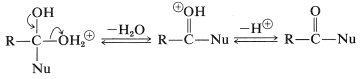An important example of this type of reaction is the formation of esters, which was discussed previously in connection with the reactions of alcohols in Section 15-4D. Similar addition-elimination mechanisms occur in many reactions at the carbonyl groups of acid derivatives. A less obvious example of addition to carboxyl groups involves hydride ion $$\left( \ce{H}^\ominus \right)$$ and takes place in lithium aluminum hydride reduction of carboxylic acids (Sections 16-4E and 18-3C).

## Esterification

Esters, $$\ce{RCO_2R'}$$, are formed from carboxylic acids and alcohols in the presence of acid catalysts. The key step in esterification is the nucleophilic attack of a neutral alcohol molecule, $$\ce{R'OH}$$, at the carbonyl carbon of the conjugate acid of the carboxylic acid, $$\ce{RC(OH)_2^+}$$, $$6$$:The intermediate, $$7$$, either can revert to the starting materials or form a second intermediate, $$8$$, by proton transfer. Loss of water from $$8$$ leads to the conjugate acid of the ester, $$9$$:The final step in formation of the ester is proton transfer from $$9$$ to the solvent:All the steps in ester formation are reversible, but the equilibrium in the $$\ce{C-O}$$ bond-making and -breaking processes are not very favorable, and an excess of one reactant (usually the alcohol) or removal of one product (most often water) is required to give a good yield of ester.

The usefulness of direct ester formation from alcohols and acids is limited to those alcohols or acids that do not undergo extensive side reactions in the presence of strong acids. Furthermore, if the alcohol is particularly bulky the reaction usually will not proceed satisfactorily because the intermediates $$7$$ and $$8$$ (as well as the product) are rendered unstable by crowding of the substituent groups.

Bulky groups in the esterifying acid also hinder the reaction. A classic example is 2,4,6-trimethylbenzoic (mesitoic) acid, which cannot be esterified readily under normal conditions because the methyl groups ortho to the carboxyl group make the transition state for formation of the intermediate $$10$$ less favorable relative to the starting acid than would be the case for less hindered acids, such as ethanoic acid:The important point is the difference in steric hindrance between the acid and the intermediate. If you make a scale model you will see that in the acid, the carboxyl group, being planar, can have reduced hindrance by turning about its bond to the ring so as to be between the methyl groups. However, no such relief is possible with $$10$$, in which the $$\ce{-C(OH)_2OR}$$ carbon is tetrahedral.

Esterification of acids with bulky substituents, such as 2,4,6-trimethylbenzoic acid, can be achieved through formation of acyl cations. This is done by simply dissolving the carboxylic acid in strong sulfuric acid, whereby the acyl cation $$11$$ is formed, and then pouring the solution into an excess of cold alcohol (see also Equations 18-5 and 18-6). This procedure works because it avoids the formation of a hindered tetrahedral intermediate similar to $$10$$ and instead forms the conjugate acid directly:Esterification of carboxylic acids with bulky alcohols is unsatisfactory. However, tertiary alkyl esters often can be prepared by addition of the acid to the appropriate alkene using an acid catalyst:The success of such addition reactions depends on formation of a stable carbocation from the alkene under conditions where the most reactive nucleophile present is the carboxylic acid.

## Acyl Chloride Formation

Carboxylic acids react with phosphorus trichloride, phosphorus pentachloride, or thionyl chloride with replacement of $$\ce{OH}$$ by $$\ce{Cl}$$ to form acyl chlorides, $$\ce{RCOCl}$$:Although detailed mechanisms have not been established, the first step is thought to be formation of an unstable mixed anhydride, which then extrudes $$\ce{SO_2}$$ and "collapses" with attach of chloride at the carbonyl carbon. A similar mechanism occurs in the formation of alkyl chlorides from alcohols and thionyl chloride (Section 15-5A):Most acyl halides are stable, distillable liquids. However, methanoyl chloride, $$\ce{HCOCl}$$, decomposes to carbon monoxide and hydrogen chloride at room temperature.

## Reduction of Carboxylic Acids

Generally, carboxylic acids are difficult to reduce either by catalytic hydrogenation or by sodium and alcohol. Nonetheless, reduction to primary alcohols proceeds smoothly with lithium aluminum hydride, $$\ce{LiAlH_4}$$: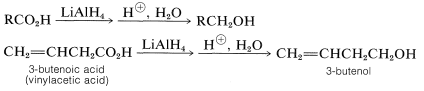The first step in lithium aluminum hydride reduction of carboxylic acids is formation of a complex aluminum salt of the acid and hydrogen: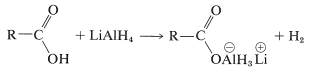Reduction then proceeds by successive transfers of hydride ion, (\ce{H}^\ominus\), from aluminum to carbon. The first such transfer reduces the acid salt to the oxidation level of the aldehyde; reduction does not stop at this point, however, but continues rapidly to the alcohol. Insufficient information is available to permit very specific structures to be written for the intermediates in the lithium aluminum hydride reduction of carboxylic acids. However, the product is a complex aluminum alkoxide, from which the alcohol is freed by hydrolysis:Sodium borohydride, $$\ce{NaBH_4}$$, is too mild a reducing agent to transfer hydride to carboxylic acids, and one may suspect that borane, $$\ce{BH_3}$$, also would be ineffective. However, this is not the case and borane in oxacyclopentane (tetrahydrofuran) reduces carboxylic acids more rapidly than it adds to alkene double bonds (see Tale 16-5):The reason for the high reactivity lies in the fact that the acid first converts the borane to an acyloxyborane, which then undergoes an intramolecular rearrangement in which the carbonyl group is reduced. Hydrolysis gives the alcohol: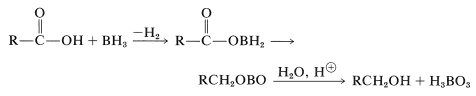Special methods are required for the direct reduction of $$\ce{RCO_2H}$$ to $$\ce{RCHO}$$. Aldehydes can be obtained directly by the slow reduction of carboxylic acids with 2,3-dimethyl-2-butylborane in oxacyclopentane solution. One hydrogen of the borane is wasted through reaction with the acidic hydrogen of the carboxyl group to give hydrogen. An example is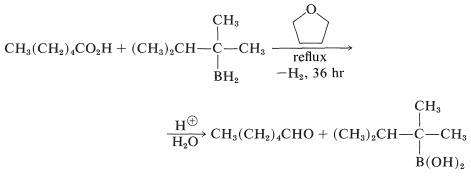The borane is prepared through the addition of $$\ce{B_2H_6}$$ to 2,3-dimethyl-2-butene and, because of steric hindrance, only the monoalkylborane is formed (Section 11-6A):